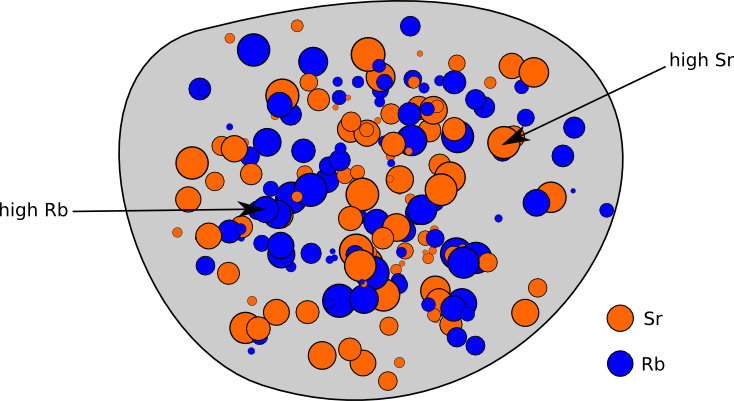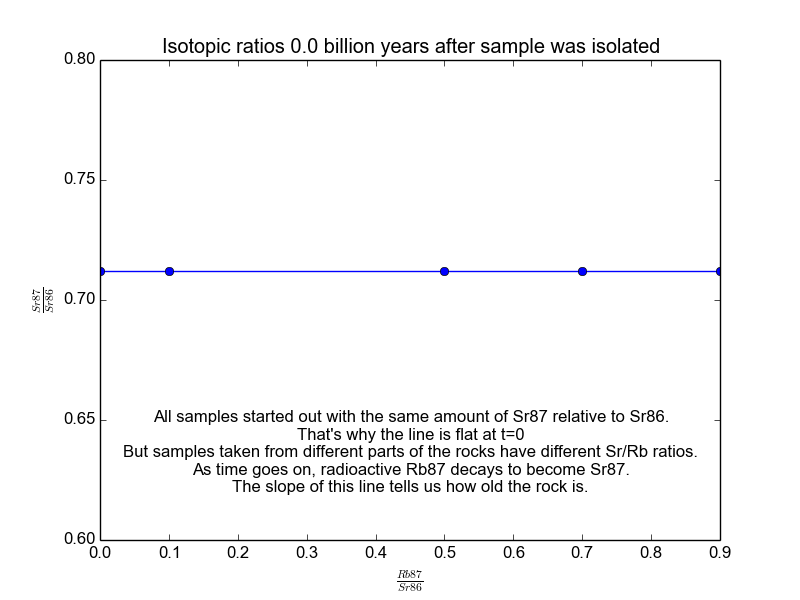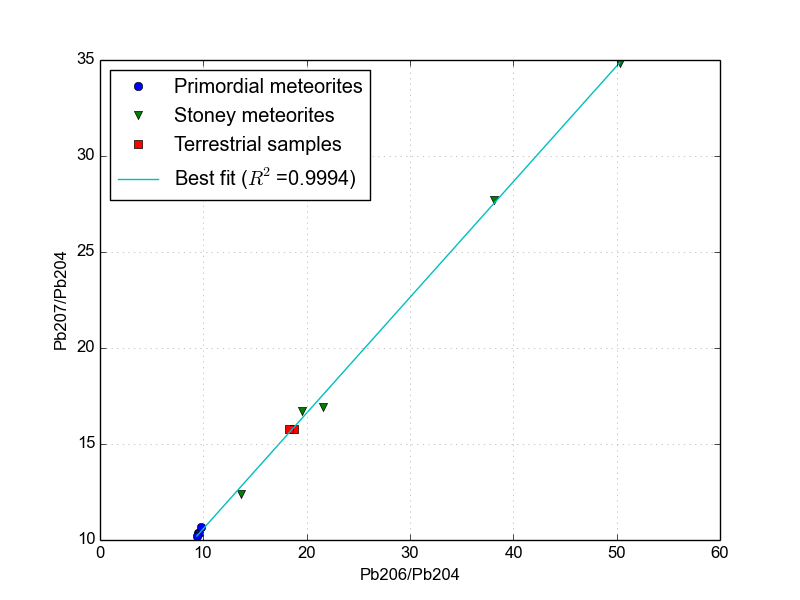# The Age of the Earth

#### By Nick Touran, Ph.D., 2014-04-27

You may have heard that the Earth is 4.54 billion years old. This was calculated by taking precise measurements of things in the dirt and in meteorites and using the principles of radioactive decay to determine an age. This page will show you how that was done.

## Fundamentals of radiogenic isotope geology

Radioactive nuclides decay with a half-life. If the half-life of a material is 100 years and you have 1 kg of it, 100 years from now you will only have 0.5 kg of it. The rest will have decayed into a different nuclide (called a daughter nuclide). Several radioactive nuclides exist in nature with half-lives long enough to be useful for geologic dating.

Let’s go through an example of calculating the age of a rock with the radioactive nuclide Rubidium-87 (Rb87). This nuclide decays to Strontium-87 (Sr87) with a half-life of 48.8 billion years. Imagine going way back in time and looking at some lava that is cooling to become a rock. It naturally has different concentrations of Rb and Sr in different parts of the rock because these don’t necessarily mix perfectly. This is shown schematically in Figure 1. Once it cools all the way and crystallizes, it is considered “born” and atoms can no longer come in or out of the system. At this point, its radiometric clock starts ticking.Figure 1. Schematic diagram of a rock with different concentrations of Rb and Sb throughout.

Though the Rb and Sr concentrations differ, it’s safe to assume that the isotopic makeup of Sr and of Rb is the same everywhere. This is the key to figuring out how much time has passed since the rock solidified. As time goes on, the Rb87 in the rock slowly turns into Sr87. Parts of the rock that have more Rb87 will end up with more Sr87. By measuring a few samples of the rock and comparing the relative amounts of Sr87 and Rb87, we can figure out how old the rock is!

The mathematics of radioactive decay shows us that the number of Sr87 nuclides that exist after some time t is: $$\text{Sr87}_{\text{now}}= \left(e^{\lambda t}-1 \right)\text{Rb87}_{\text{now}} +\text{Sr87}_{\text{orig}}$$

We can measure $$\text{Sr87}_{\text{now}}$$, $$\text{Rb87}_{\text{now}}$$, and $$\lambda$$, but we can’t measure $$\text{Sr87}_{\text{orig}}$$ (no one was around to measure it back then). So what do we do? We use something called an isochron. If you think about it, the equation above is a lot like the formula for a line, $$y=mx+b$$ with $$y=\text{Sr87}_{\text{now}}$$, $$m= \left(e^{\lambda t}-1 \right)$$, $$x=\text{Rb87}_{\text{now}}$$, and $$b=\text{Sr87}_{\text{orig}}$$. Because the rock originally had different mixtures of Rb and Sr, we can expect to get different points for each sample we measure, and if all samples have the same age, then we expect to see a straight line (hence the name isochron).

We plot all our measurements and then fit a line through them. The slope of the line can then be solved for $$t$$, giving us the age of the rock. As a bonus, the intercept ($$b$$) of the line tells us the value of $$\text{Sr87}_{\text{orig}}$$ because we know the line was flat when the age of the rock was zero. The animation in Figure 2 shows the flat line and how it increases with time.Figure 2. The isochrons of the Rb/Sr clock. Note that the values of the axes are actually normalized by Sr86 because the mass spectrometers used to take these measurements are much more accurate at relative values than they are at absolutes. It works because Sr86 is stable and not radiogenic and therefore stays constant with time.

The isochron method can determine the age of any rock, but new rocks are formed all the time. So to figure out the age of the Earth, we have to look somewhere else… in the sky!

## The age of the Earth

Earth has a molten magma layer and plate tectonics, so the “closed system” requirement of these radiometric dating methods is sometimes difficult to satisfy for Earth itself. Meteorites, on the other hand, have been floating around in space since the solar system was formed. When they come crashing to Earth, analysis of their composition can be geologically analyzed.

Claire Patterson was the first to accurately date the crystallization of Earth to 4.55 +/- 0.05 billion years ago. He used a lead isotope isochron method using measurements from three different meteorites (lead-206, lead-207 are the eventual decay products of uranium-238 and uranium-235). He then took measurements from the deep ocean that fell squarely on the meteorite isochron, suggesting that the Earth and the meteorites were both created at the same time, 4.55 billion years ago. (You may have learned from COSMOS that he also was among the first to understand the public health risks of lead contamination from leaded gasoline).

Many other methods have been used to date the Earth, with many different sets of radioactive nuclides (and other methods). They are all consistent with Patterson’s measurement. This is how we know how old the Earth is.

The mathematical details of the lead-lead isotopic clock are less straightforward than those of the Rb-Sr method. On the other hand, since only lead is involved (instead of two chemical species), the lead-lead clock is resilient against situations where the samples were recently weathered or otherwise “opened”. We will skip the isochron derivation, but you can find it elsewhere. The end result is that the slope of the isochron with Pb207 concentrations graphed against Pb206 (both relative to non-radiogenic Pb204) is equal to:

$m = \frac{N_{U235}}{N_{U238}} \frac{e^{\lambda_{U235} t}-1}{e^{\lambda_{U238} t}-1}$

Data from several meteorites and from a few terrestrial sources are shown in Figure 3. The data have been replotted from the tables of .Figure 3.The Pb-Pb isochron of several meteorites and deep Earth samples. Since this determines the age of the Earth, it is also known as the Geochron.

The best-fit line shown in Figure 3 has a slope of 0.602459. Unfortunately, the geochron equation above is transcendental, meaning there’s no algebraic solution for it and it has to be solved numerically. Conveniently, this is very trivial with a few lines of Python. Using this python program that I wrote, I am able to instantly calculate the age of the Earth to be 4.51 billion years using lead isotopic measurements alone. Cool! This is within the uncertainty claimed by Patterson.

Note that Uranium-235 decays to Lead-207 and that Uranium-238 decays to Lead-206. There are short-lived details in these decay chains, but they don’t matter on the timescales we’re dealing with. See the decay chain link in the See Also section below for decay chain details.

## Carbon dating

Carbon dating is often discussed. It involves the radioactive nuclide Carbon-14 (aka radiocarbon) decaying to Nitrogen-14 with a 5730 year half-life. As you can imagine, this half-life is short with respect to the age of the Earth, so it can’t be used to date rocks. But, it is unmatched in its ability to date things that used to be living (bones, wood, food, paper, etc.). When a living thing dies, its body stops uptaking carbon. This is analogous to a rock crystallizing, as the body now becomes a closed system of carbon, and so a radiocarbon clock starts in it. Radiocarbon dating has been used for living things in the 100,000 year timeframe. Find out more about it through our See Also links below.

## Age of the Universe

All the discussions on this page so far relate to the age of rocks and of the Earth. But this is not the same as the age of the universe. Scientists have found much evidence for The Big Bang that created the universe 13.8 billion years ago. This created all the building blocks of atoms. Since then, the atoms, both large and small have been forged in stars in the processes of stellar nucleosynthesis.

There are certainly some assumptions made to perform these calculations. Let’s go through some of them.

## The half-lives of radioactive decay have remained constant since the formation of the Earth

This assumption, also known as Uniformitarianism, needs to be justified before considering the calculations on this page accurate. If the half-lives change, then we’re out of luck. Fortunately, there is a lot of evidence that they don’t change. The simplest of which, perhaps is that geologic clocks from 5 or 6 different nuclide pairs give consistent results for the same samples. If half-lives were changing, it’d be an unbelievable coincidence that these independent methods agree so well.

Another fun fact is that 2 billion years ago, conditions in Africa were such that a natural nuclear reactor went critical at Oklo. The abundances of fission products from this reactor are measurable today, and they are consistent with constant half-lives. So this at leasts tells us that the half-lives were constant for the past 2 billion years.

## The isotopic abundances were uniform throughout the solar system in the beginning

The fact that lunar, meteorite, and terrestrial samples all have consistent isotopic distributions for the most part strongly suggests that this is true.

1. The mathematics of radioactive decay